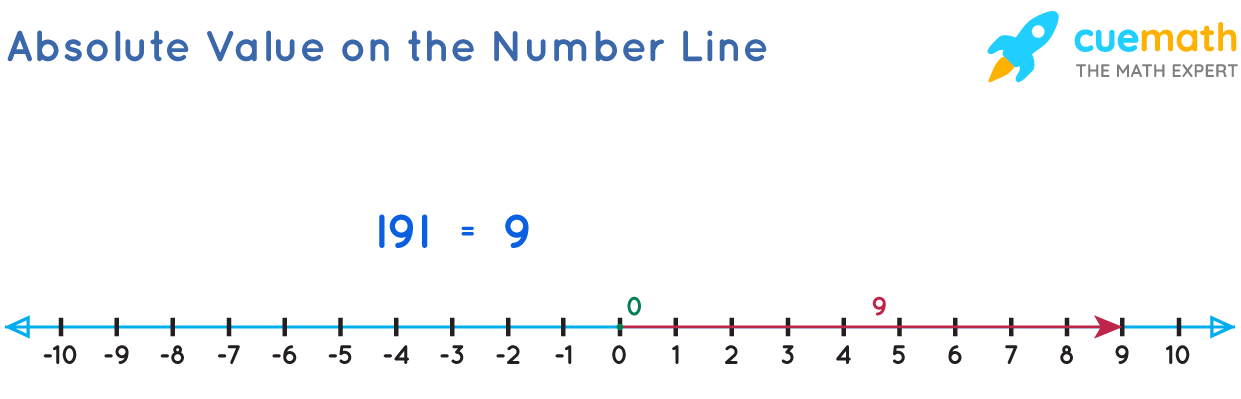# What Is the Absolute Value of 9

## Question: What is the absolute value of 9?

The absolute value of a variable x is represented by |x| which is pronounced as "Mod x" or "Modulus of x."

## Answer: The absolute value of 9 =  |9| = 9

Other names of "Absolute Value" are "Numerical value" and "Magnitude".

## Explanation:

The absolute value of a number is its distance from 0. The absolute value of a number is the magnitude of that number without considering its sign.

The absolute value of 9 is written as |9| and is equal to 9.